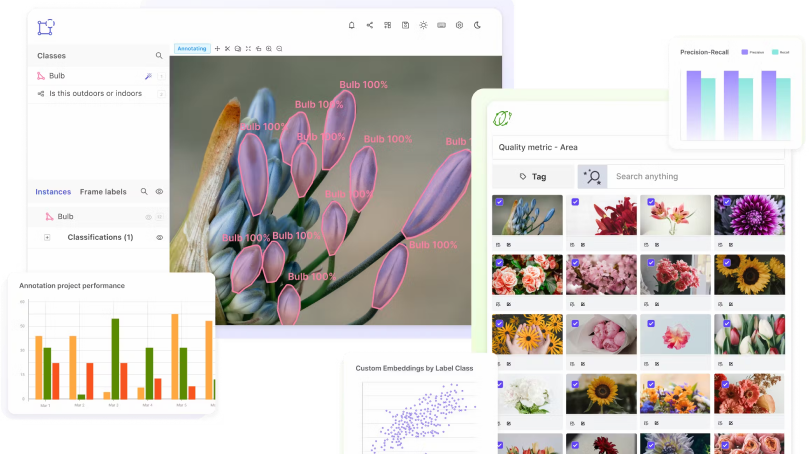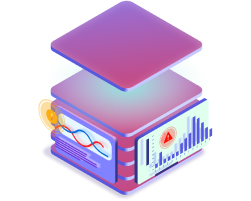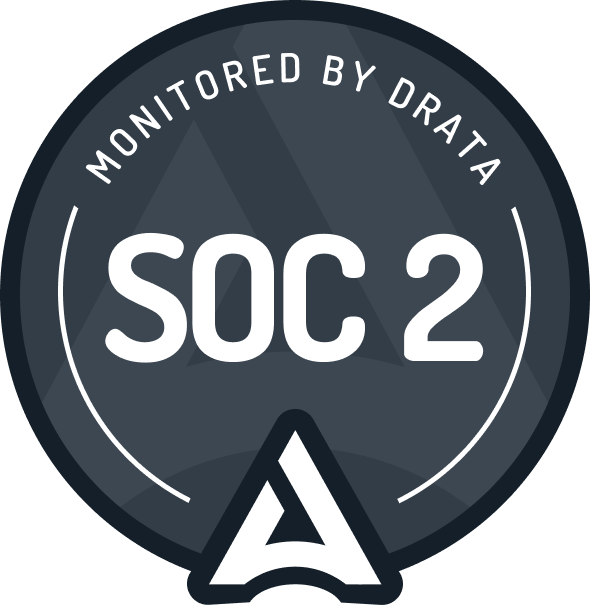# Supervised Learning

Encord Computer Vision Glossary

## Supervised learning

Supervised learning is a type of machine learning (ML) in which a model is trained on labeled data to make predictions or decisions. It is called "supervised" because the data used to train the model includes both input data and the corresponding correct output labels.

Supervised learning algorithms are used in a variety of applications, including image classification, natural language processing, and predictive modeling. They are able to learn from the labeled data and make predictions or decisions about new, unseen data.

There are two main types of supervised learning: classification and regression. Classification algorithms are used to predict a categorical outcome, such as whether an email is spam or not spam. Regression algorithms are used to predict a continuous outcome, such as the price of a stock.

As the right output labels are provided with the input data, supervised learning has the major benefit of using relatively minimal data to train the model. To make sure that the model can generalize to fresh, untested data, it is crucial to have a suitably sizable and varied dataset.\

The two types of supervised learning algorithms are linear and nonlinear, respectively. Non-linear algorithms are based on relationships between the input and output data that are more sophisticated than those of linear algorithms and assume a non-linear relationship between the input and output data.

Overall, supervised learning is a type of ML in which a model is trained on labeled data to make predictions or decisions. It is widely used in a variety of applications, and is able to learn from the labeled data and make predictions or decisions about new, unseen data.

Scale your annotation workflows and power your model performance with data-driven insights## How is supervised learning used for computer vision?

Supervised learning algorithms are used in a variety of applications, including image classification, natural language processing, and predictive modeling. They are able to learn from the labeled data and make predictions or decisions about new, unseen data.

There are two main types of supervised learning: classification and regression. Classification algorithms are used to predict a categorical outcome, such as whether an email is spam or not spam. Regression algorithms are used to predict a continuous outcome, such as the price of a stock.

As the right output labels are provided with the input data, supervised learning has the major benefit of using relatively minimal data to train the model. To make sure that the model can generalize to fresh, untested data, it is crucial to have a suitably sizable and varied dataset.

The two types of supervised learning algorithms are linear and nonlinear, respectively. Non-linear algorithms are based on relationships between the input and output data that are more sophisticated than those of linear algorithms and assume a non-linear relationship between the input and output data.

Overall, supervised learning is a type of ML in which a model is trained on labeled data to make predictions or decisions. It is widely used in a variety of applications, and is able to learn from the labeled data and make predictions or decisions about new, unseen data.

#### The Complete Data Engine for AI Model Development###### Subscribe